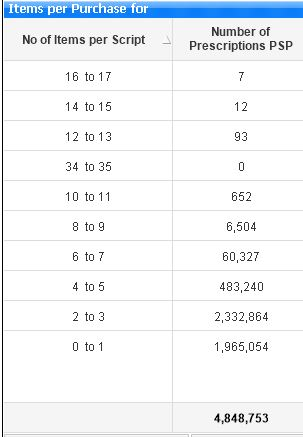New to QlikView

Discussion board where members can get started with QlikView.

Valued Contributor

Class Sorting

I am creating a calculated dimension using the following to determine the "No of Items per Script" class dimension:

=Dual(Left(Aggr(Class(Count([Drug Name]), 2), [Script Number]), FindOneOf(Aggr(Class(Count([Drug Name]), 2), [Script Number]), ' ',1))
& ' to ' &
(
Num(Mid(Aggr(Class(Count([Drug Name]),2), [Script Number]),FindOneOf(Aggr(Class(Count([Drug Name]), 2), [Script Number]),' ',4)))-1), Left(Aggr(Class(Count([Drug Name]), 2), [Script Number]), FindOneOf(Aggr(Class(Count([Drug Name]), 2), [Script Number]), ' ',1))
& ' to ' &
(
Num(Mid(Aggr(Class(Count([Drug Name]),2), [Script Number]),FindOneOf(Aggr(Class(Count([Drug Name]), 2), [Script Number]
),' ',4)))-1))

My chart looks as follows:I then sort by Ascending value based on "No of Items per Script" but the chart does not sort properly as shown above.  Please provide a solution to this.

Regards.

Chris

Tags (2)
1 Solution

Accepted Solutions
Valued Contributor II

Re: Class Sorting

Try putting the below expression in the Sorting tab under the Expression and sort by ascending

Left(Aggr(Class(Count([Drug Name]), 2), [Script Number]), FindOneOf(Aggr(Class(Count([Drug Name]), 2), [Script Number]), ' ',1))

8 Replies
Honored Contributor III

Re: Class Sorting

or put your expression in Sort >> sort by expression >> ascMVP & Luminary

Re: Class Sorting

Try it without the dual() because class() is per default a dual-value - you could easily check it if you just wrap your class() with a num(). To change the interpretation you could adjust the 3. parameter of the function.

- Marcus

Valued Contributor II

Re: Class Sorting

Try putting the below expression in the Sorting tab under the Expression and sort by ascending

Left(Aggr(Class(Count([Drug Name]), 2), [Script Number]), FindOneOf(Aggr(Class(Count([Drug Name]), 2), [Script Number]), ' ',1))

Valued Contributor

Re: Class Sorting

Thank you PM.  I changed the function to:

=Left(Aggr(Class(Count([Drug Name]), 2), [Script Number]), FindOneOf(Aggr(Class(Count([Drug Name]), 2), [Script Number]), ' ',1))
& ' to ' &
(
Num(Mid(Aggr(Class(Count([Drug Name]),2), [Script Number]),FindOneOf(Aggr(Class(Count([Drug Name]), 2), [Script Number]),' ',4)))-1)

As mentioned by Marcus, class is a dual by default.  I then using the above expression to sort by descending and i get the following:There are still some anomalies on the sort order where the range 34 to 35 is misplaced.

Regards.

Chris

Valued Contributor

Re: Class Sorting

Thanks, Susant, most appreciated.

Regards.

Chris

Valued Contributor

Re: Class Sorting

Thank you Marcus for your contribution, most appreciated.

Regards.

Chris

Highlighted
Honored Contributor III

Re: Class Sorting

Hi Christopher,

if You want replace >= <= with 'to', then You can use Floor() function instead, like thisRegards,

Antonio

Valued Contributor